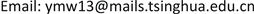1中国人民大学附属中学，北京

2清华大学航天航空学院，北京1. 引言

2. 太空站位置与电梯长度的分析2.1. 太空站在地球同步轨道上

F 1 = ∫ R e R 0 + L G M λ d r r 2 (1)

F 2 = ∫ R e R 0 + L ω 2 r λ d r (2)

ω 2 R 0 = G M R 0 2 (3)

L = 2 R 0 3 R e + 1 4 R e 2 − 1 2 R e − R 0 (4)

F 1 = ∫ R e R 0 G M λ 1 d r r 2 + ∫ R 0 R 0 + L G M λ 2 d r r 2 (5)

F 2 = ∫ R e R 0 ω 2 r λ 1 d r + ∫ R 0 R 0 + L ω 2 r λ 2 d r (6)

(7)

2.2. 太空站不在地球同步轨道上

F 3 = m ( ω 2 R 0 − G M R 0 2 ) (8)

1 2 k L 3 + 3 2 k R 0 L 2 + [ k R 0 2 + 1 2 ( R 0 2 − R e 2 ) + R s ( R 0 − R G 3 R 0 2 ) − R G 3 R e − k R G R 0 + R G 3 R 0 ] L + 1 2 ( R 0 2 − R e 2 ) R 0 + R s ( R 0 − R G 3 R 0 2 ) R 0 + R G 3 − R G 3 R 0 R e = 0 (9)

3. 太空电梯径向方向应力分布

F ( R ) = { ∫ R e R ( G M r 2 − ω 2 r ) λ 1 d r ,                                                                                                                             ( R < R 0 ) ∫ R e R 0 ( G M r 2 − ω 2 r ) λ 1 d r + ∫ R 0 R ( G M r 2 − ω 2 r ) λ 2 d r + m ( G M R 0 2 − ω 2 R 0 ) ,     ( R > R 0 ) (10)

σ ( R ) = { ρ 1 ω 2 [ R G 3 R e − R G 3 R − 1 2 ( R 2 − R e 2 ) ] ,                                                                                                                                           ( R < R 0 ) ρ 1 ω 2 { [ R G 3 R e − R G 3 R 0 − 1 2 ( R 0 2 − R e 2 ) ] + k [ R G 3 R 0 − R G 3 R − 1 2 ( R 2 − R 0 2 ) ] + R s ( R G 3 R 0 2 − R 0 ) } ,     ( R > R 0 ) (11)

1) 太空站布置在地球同步轨道上，两端电梯采用相同材料( R 0 = R G , k = 1 )；

2) 太空站布置在地球同步轨道上，两端电梯采用不同材料( R 0 = R G , k = 2.82 )；

3) 太空站布置在8.36Re上，两端电梯采用相同材料( R 0 = 8.36 R e , k = 1 )；

4) 太空站布置在8.36Re上，两端电梯采用不同材料( R 0 = 8.36 R e , k = 2.82 )。

4. 电梯运送载荷的特性分析4.1. 单位质量载荷运送至太空站所需能量的计算

Δ φ 1 + Δ φ 2 = G M R e − G M R 0 − 1 2 ω 2 ( R 0 2 − R e 2 ) (12)

W min = G M R e − G M R 0 − 1 2 ω 2 ( R 0 2 − R e 2 ) (13)

4.2. 电梯运送载荷时太空站控制所需冲量

I = ∫ t ( R e ) t ( R 0 ) r − R e R 0 − R e ⋅ 2 ω v d t = 2 ω ∫ R e R 0 r − R e R 0 − R e d r = ω ( R 0 − R e ) (14)

5. 结论

1) 通过增加太空电梯配重段的线密度，可以减小电梯的总长度；

2) 对于一定的电梯材料线密度比，在比地球同步轨道更高的轨道上，存在一个太空站的位置，使得电梯总长度最小；

3) 在地球同步轨道高度的位置，太空电梯所受到的拉力最大；对于碳纳米管材料，该处的应力约为77.5 GPa；

4) 载荷运送至太空站所需能量以及运送过程中太空站所需要的控制冲量，仅与太空站的位置有关，且太空站轨道高度越低，所需的控制冲量越小。